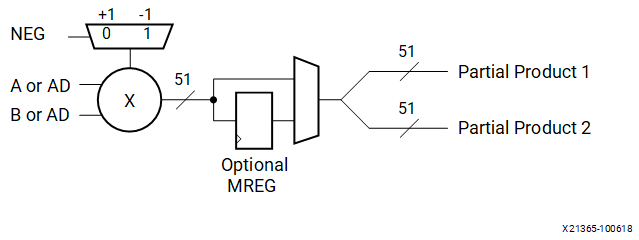# Two’s Complement Multiplier

## Versal ACAP DSP Engine Architecture Manual (AM004)

Document ID
AM004
Release Date
2022-09-11
Revision
1.2.1 English

The two’s complement multiplier in DSP58 in Figure 1 accepts a 27-bit two’s complement input and a 24-bit two’s complement input along with a conditional product negation control input bit. The multiplier produces two 51-bit partial products. The two partial products together give an 51-bit result at the output of the multiplier, as shown in the following figure.

Note: The product-negation input does not cause overflow and is safe to use for all values of the multiplier inputs. Specifically, an N-bit two’s complement number is in the range ```[–2N-1, 2N – 1 – 1]```.

All values in this range can be negated to fit within N bits except ```–2N – 1 ```, the most negative integer. But the DSP58 negates the product, not the inputs. The output of the multiplier has an inversion of the sign if `Sign(A) != Sign(B)`. The indicator makes the inputs appear to have grown but a wider multiplier is unnecessary to avoid overflow. As a result overflow can be avoided even if one or both of the multiplier inputs are the most negative numbers. The DSP58 multiplier can compute the absolute value of a two’s complement number up to 27 bits. Define the DSP58 multiplier as `mult(A[26:0],[B[23:0], p) ≡ (–1)p AB` , where `A` and `B` are the 27- and 24-bit inputs, and `p` is the Boolean product-negation control input. Then the absolute value of `A` can be computed as ```mult(A[26:0], 1, A)```.

Cascading of multipliers to achieve larger products is supported with a 23-bit, right-shifted, cascaded output bus. The right shift is used to right justify the partial products by the correct number of bits. This cascade path feeds into the Z multiplexer, which is connected to the adder/subtracter of an adjacent DSP58. The multiplier can emulate unsigned math by setting the MSB of an input operand to zero.

The following figure shows an optional pipeline register (MREG) for the output of the multiplier. Using the register provides increased performance with an increase of one clock latency.

Figure 1. Two’s Complement Multiplier Followed by Optional MREG